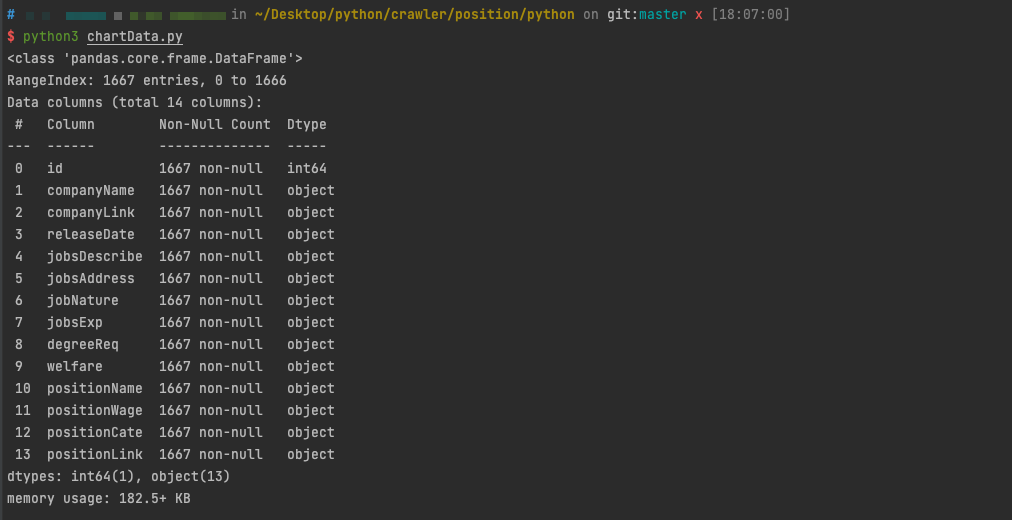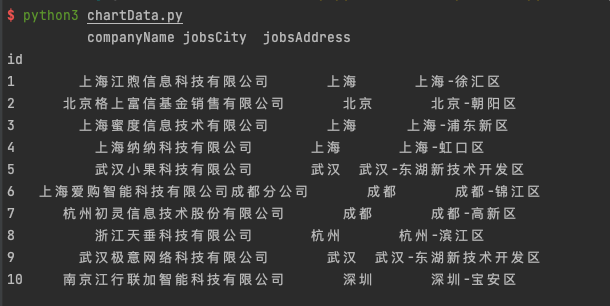# 某勾数据分析系列(二)之数据清洗

### 環境

python 3.9.0+
macos Big Sur 11.2.3+

python包 : pandas,re

### 正文

python的re包是自帶包，该包中提供的很多功能是基于正则表达式实现的，而正则表达式是对字符串进行模糊匹配，提取自己需要的字符串部分
Pandas就更加厉害了，该包是为了解决数据分析的功能而出现的。Pandas 纳入了大量库和一些标准的数据模型，有效的提供了操作大型数据集所需的方法。用了他之后你很快就会发现，它是使Python成为强大而高效的数据分析环境的重要因素之一。

#### 获取数据集合的信息

``````if __name__ == '__main__':
data.info()``````

pandas把所有数据读取之后，通过info()函数可以获取这个数据集的信息#### 生成Excel表格

``````if __name__ == '__main__':

data.to_excel('pythonData.xls')``````

``````if __name__ == '__main__':

data.index = data['id']
del(data['id'])

data.to_excel('pythonData1.xls')``````#### 数据清洗

``````import pandas
import re

class Draw:
def __init__(self):

'''
重組排序規則，使用自己的id作為索引
'''
self.data.index = self.data['id']
del (self.data['id'])
dfSort = self.data.sort_index()
self.data = dfSort

def cityDataRinse(self):
# 正则匹配字符 - 前面的所有字
pattern = re.compile('(.*?)(\-)')

for i in range(len(dfCity)):
item = dfCity.iloc[i].strip()
result = re.search(pattern2, item)
if result:
dfCity.iloc[i] = result.group(1).strip()
else:
dfCity.iloc[i] = item.strip()

self.data['jobsCity'] = dfCity

return self.data
if __name__ == '__main__':

draw = Draw()
data = draw.cityDataRinse()
print(res)``````----------end

tag(s): 数据
show comments · back · home